## Coalgebras, Comonads, and Distributive Laws

Updated: 2016-11-19

In my current project investigating the categorical semantics of attack trees we are using distributive laws to compose comonads that model various structural rules – weakening, contraction, exchange, etc – in linear logic. During the QA section of my talk at Linear logic, mathematics and computer science – see my post on my talk and the workshop for more details – Paul-André Melliès suggested using adjunctions to compose the structural rules instead of distributive laws for the obvious reason that they really compose as opposed to monads/comonads. I told him that I did try to this initially, but was not able to get it working. He was nice enough to talk with me a bit and gave me some hints to how it works which are based on a paper of his.

Instead of just looking up the details in his paper I wanted to see if I could recreate his result with his hints. I often do this for learning purposes. So that’s what I did during my flight back from France. I figured why not make it a blog post. Keep in mind that this result is due to Paul-André, but I had a lot of fun recreating his steps.

I plan to write a follow up post describing how this result can be used to to create various adjoint models of linear logic, but here we show that when we have two comonads on a category with a distributive law, then those comonads in addition to their composition can be decomposed into a composition of adjunctions between their coalgebras.

Suppose $(k,\varepsilon,\delta)$ is a comonad on a category $\cat{L}$. Then it is well known that it can be decomposed into the following adjunction: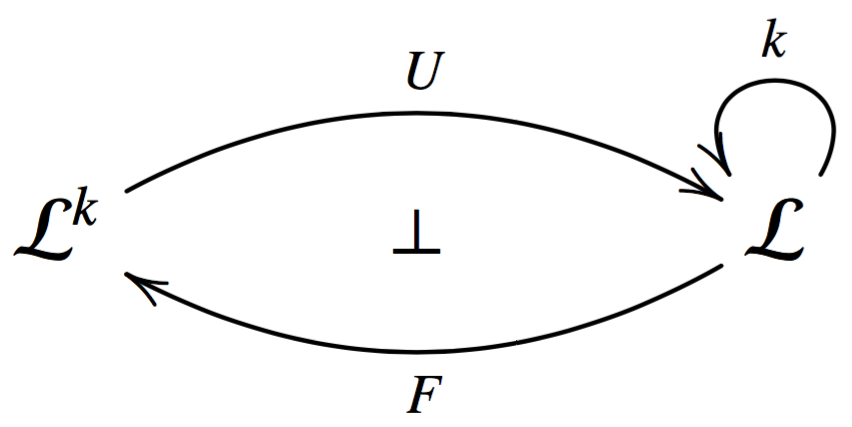where $U : \cat{L}^k \mto \cat{L}$ is the forgetful functor, $F : \cat{L} \mto \cat{L}^k$ is the free functor, and $k = UF : \cat{L} \mto \cat{L}$.

Now suppose we have the following adjunctions: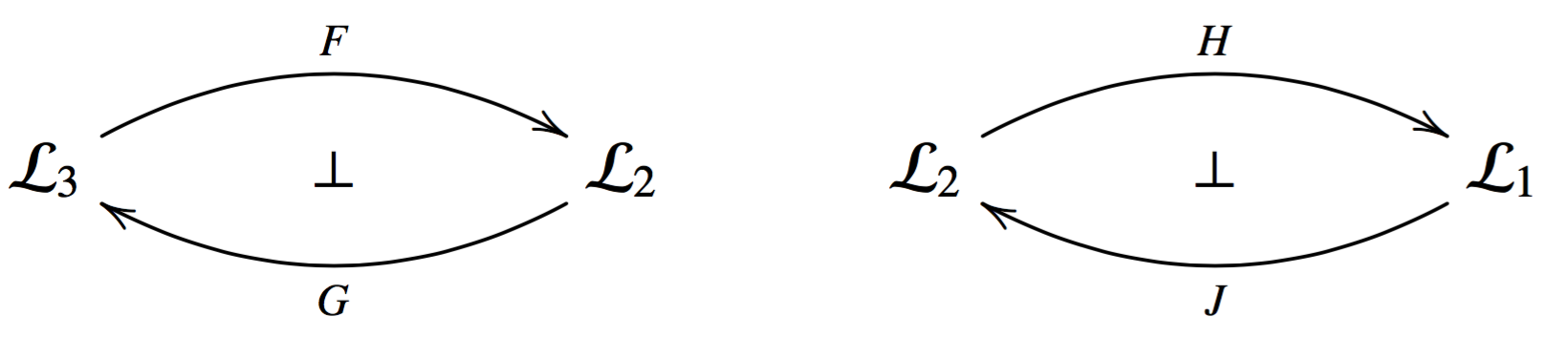Then they can be composed into the adjunction: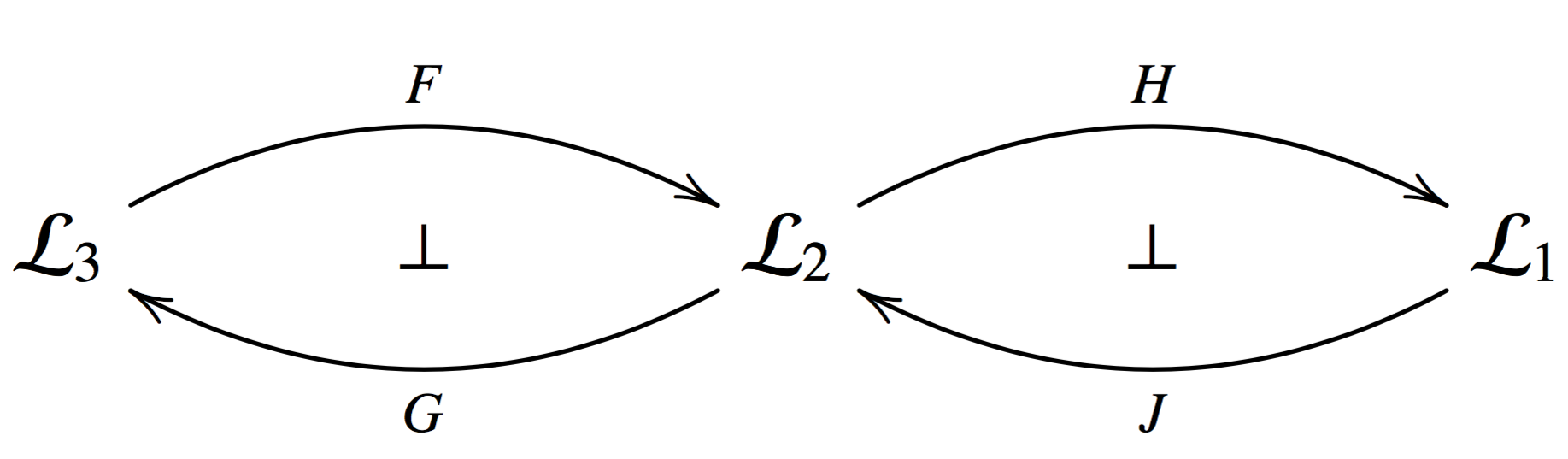Keep in mind that this gives rise to a comonad $HFGJ : \cat{L}_1 \mto \cat{L}_1$.

We are going to use these two facts to compose comonads using adjunctions. Suppose we have the comonads $(k_1,\varepsilon^1,\delta^1)$ and $(k_2,\varepsilon^2,\delta^2)$ both on a category $\cat{L}$ with a distributive law – distributive laws for comonads are defined to be the opposite of the distributive laws for monads$\mathsf{dist} : k_2k_1 \mto k_1k_2$. Thus, making $k_2k_1 : \cat{L} \mto \cat{L}$ a comonad.

Then we can decompose $k_1$ into a an adjunction: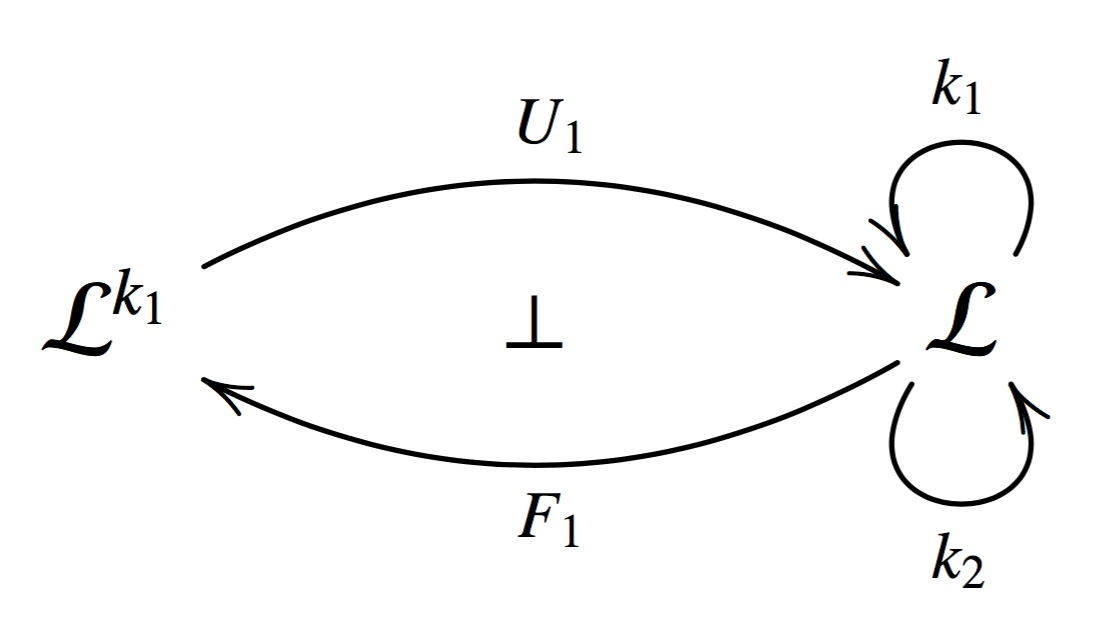Here we know that $k_1 = U_1F_1 : \cat{L} \mto \cat{L}$, but we also know something about $k_2$. We can extend it to a comonad on $\cat{L}^{k_1}$.

First, we define the functor $\widetilde{k}_2 : \cat{L}^{k_1} \mto \cat{L}^{k_1}$ to send objects $(A,h_A)$ to $(k_2A,h'_A)$, where $h'_A := k_2A \mto^{k_2h_A} k_2k_1 A \mto^{\mathsf{dist}_A} k_1k_2 A$, and to send morphisms $f : (A,h_A) \mto (B,h_B)$ to the morphism $k_2f : (k_2A,h'_A) \mto (k_2B,h'_B)$. We must show that $k_2f : k_2A \mto k_2B$ is a coalgebra morphism, but the following diagram commutes: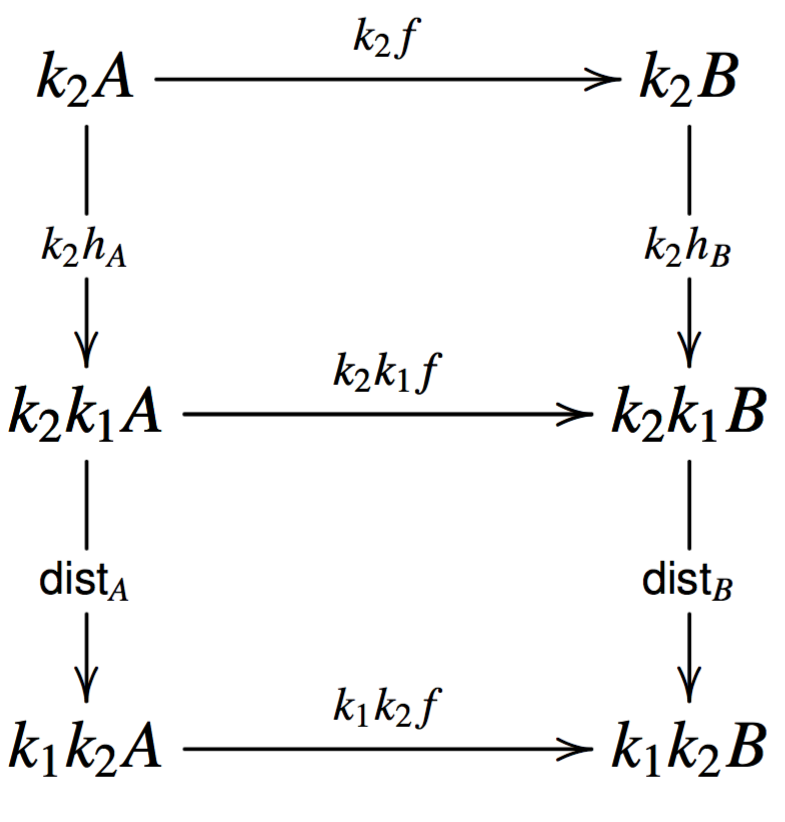The top diagram commutes because $f$ is a coalgebra morphism and the bottom diagram commutes by naturality of $\mathsf{dist}$. Since the morphism part of $\widetilde{k}_2$ is defined using the functor $k_2$ we know $\widetilde{k}_2$ will respect composition and identities.

We now must show that $\widetilde{k}_2$ is a comonad. The natural transformation $\widetilde{\varepsilon^2} : \widetilde{k}_2 \mto \mathsf{Id}$ has components $\widetilde{\varepsilon^2}_{(A,h_A)} = \varepsilon^2_A : \widetilde{k}_2 (A,h_A) \mto (A,h_A)$. We must show that $\varepsilon^2_A$ is a coalgebra morphism between $\widetilde{k}_2 (A,h_A) = (k_2A,k_2h_A;\mathsf{dist}_A)$ and $(A,h_A)$, but this follows from the following diagram: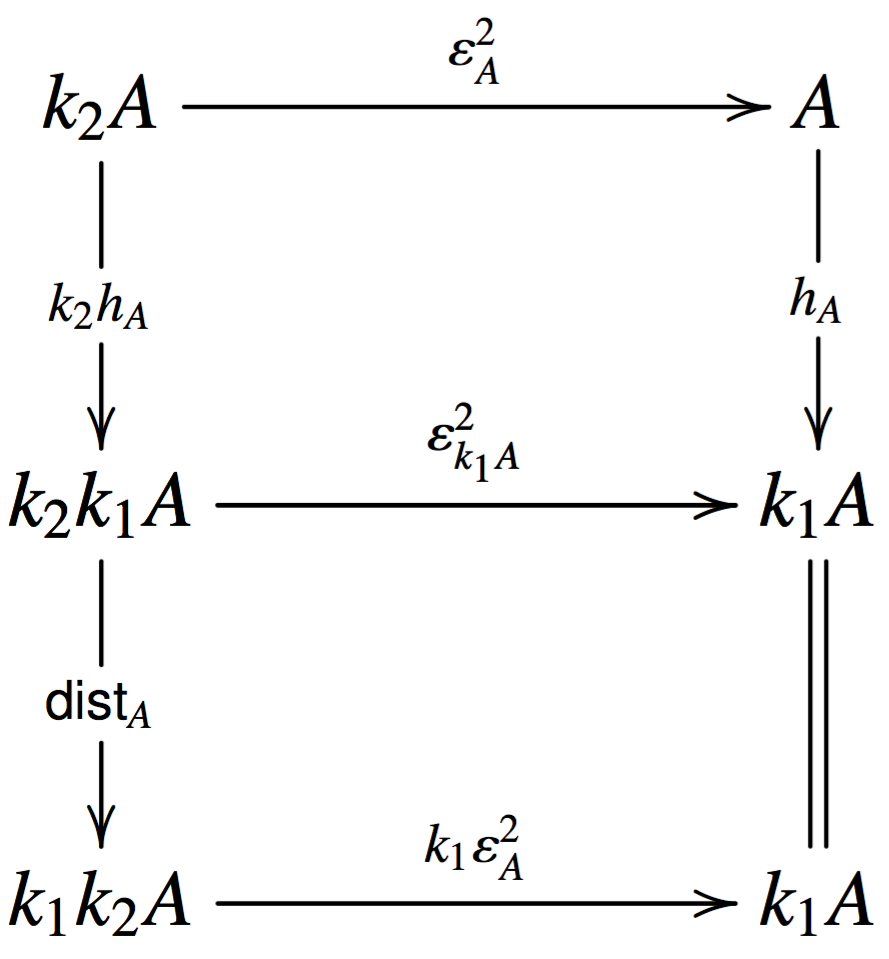The top diagram commutes by naturality of $\varepsilon^2$ and the bottom diagram commutes by the conditions of the distributive law. Naturality for $\widetilde{\varepsilon^2}$ easily follows from the fact that it is defined to be $\varepsilon_2$.

The natural transformation $\widetilde{\delta^2} : \widetilde{k_2} \mto \widetilde{k_2}\widetilde{k_2}$ has components $\widetilde{\delta^2}_{(A,h_A)} = \delta^2_A : \widetilde{k_2}(A,h_A) \mto \widetilde{k_2}\widetilde{k_2}(A,h_A)$. Just as above we must show that $\delta^2_A : k_2A \mto k^2_2 A$ is a coalgebra morphism between $\widetilde{k_2}(A,h_A) = (k_2A,k_2h_A;\mathsf{dist}_A)$ and $\widetilde{k_2}\widetilde{k_2}(A,h_A) = (k^2_2A,k^2h_A;k_2\mathsf{dist}_A;\mathsf{dist}_{k_2A})$, but this follows from the following diagram: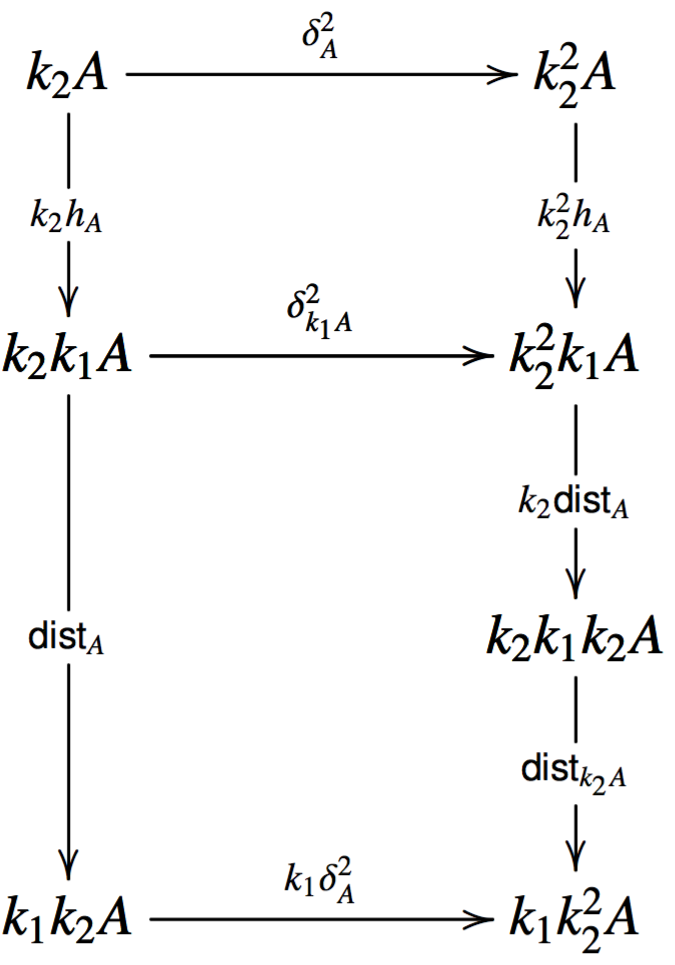The top diagram commutes by naturality of $\delta^2$ and the bottom diagram commutes by the conditions of the distributive law.

It is now easy to see that $\widetilde{\varepsilon^2}$ and $\widetilde{\delta^2}$ make $\widetilde{k_2}$ a comonad on $\cat{L}^{k_1}$, because the conditions of a comonad will be inherited from the fact that $\varepsilon^2$ and $\delta^2$ define a comonad on $\cat{L}$.

At this point we have arrived at the following situation: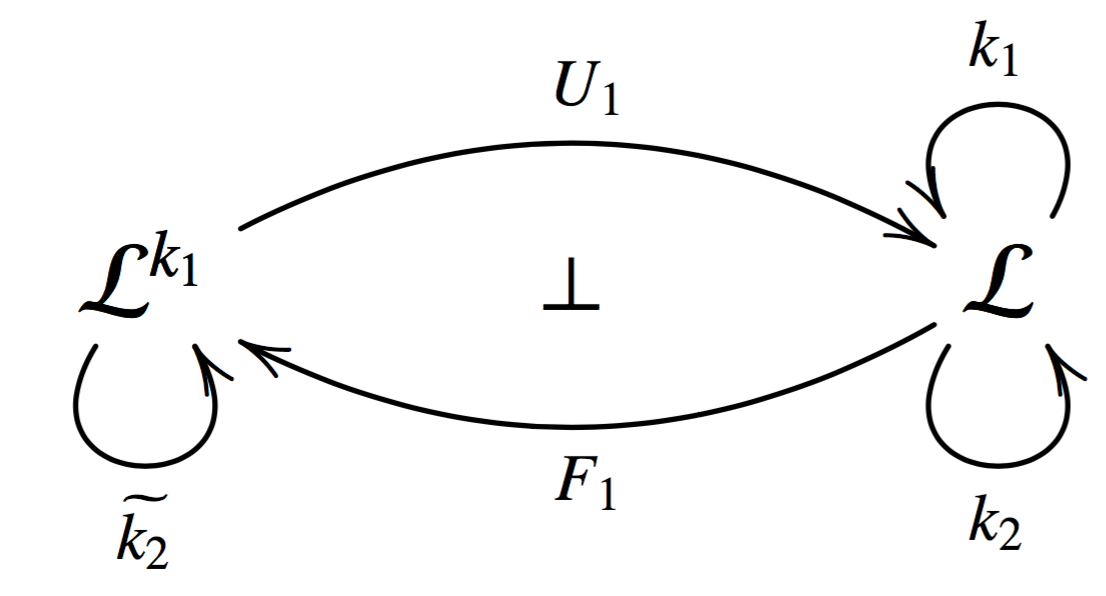Since we have a comonad $\widetilde{k_2} : \cat{L}^{k_1} \mto \cat{L}^{k_1}$ we can form the following adjunction: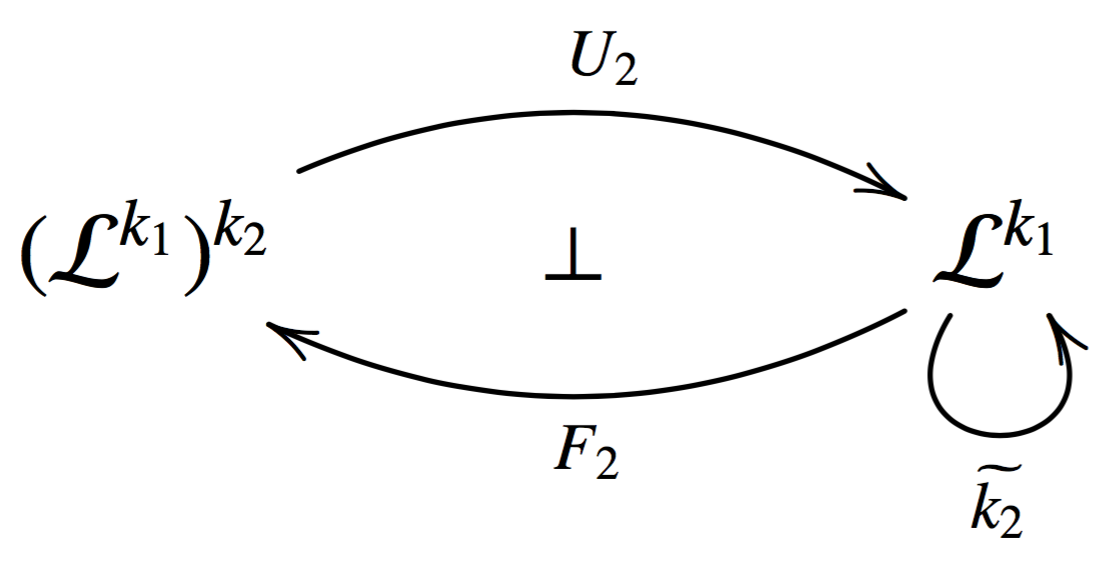The functor $F_2(A,h_A) = (\widetilde{k_2}(A,h_A), \widetilde{\delta^2}_{(A,h_A)})$ is the free functor, and $U_2(A,h_A) = A$ is the forgetful functor. Thus, we can think of $(\cat{L}^{k_1})^{k_2}$ as the world with all the structure of $\cat{L}$ extended with all of the structure $k_1$ brings and all the structure $k_2$ brings. That is, $(\cat{L}^{k_1})^{k_2}$ is the algebras of $k_2k_1 : \cat{L} \mto \cat{L}$.

We can see that the previous two adjunctions compose: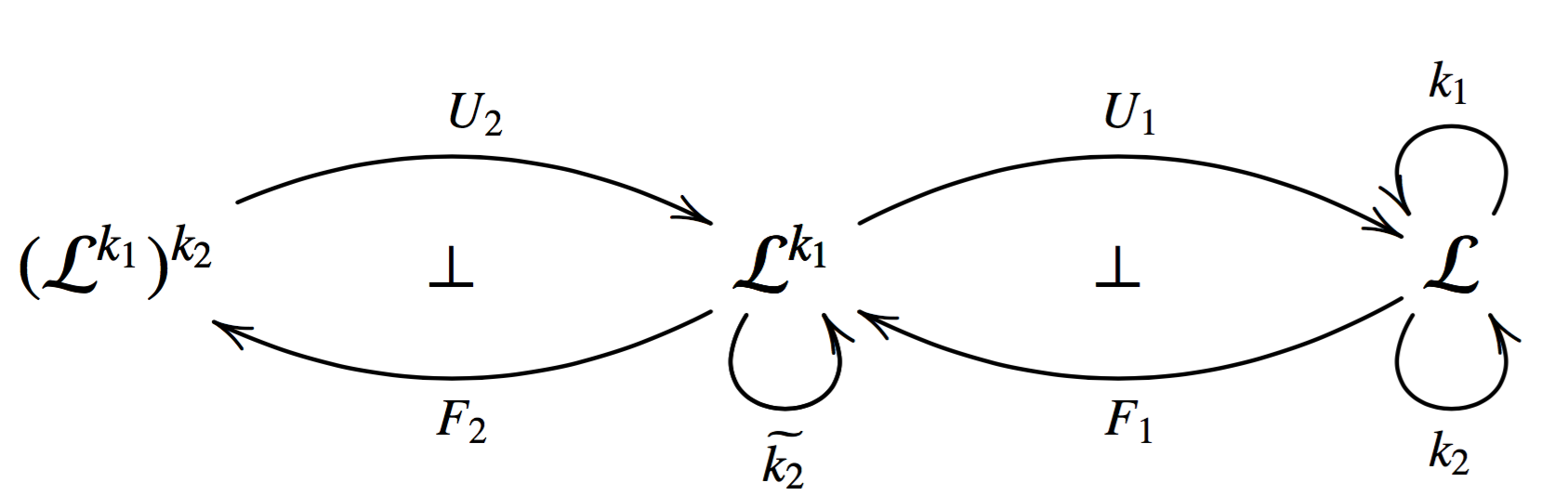Thus, we have a comonad $U_1U_2F_2F_1 : \cat{L} \mto \cat{L}$. Chasing an object through this comonad yields the following:
$\begin{array}{lll} U_1U_2F_2F_1A & = & U_1U_2F_2(k_1A,\delta^1_A)\\ & = & U_1U_2(\widetilde{k_2}((k_1A,\delta^1_A)), \widetilde{\delta^2}_{(k_1A,\delta^1_A)})\\ & = & U_1U_2((k_2k_1A,k_2\delta^1_A;\mathsf{dist}_{k_1 A}), \widetilde{\delta^2}_{(k_1A,\delta^1_A)})\\ & = & U_1(k_2k_1A,k_2\delta^1_A;\mathsf{dist}_{k_1 A})\\ & = & k_2k_1A\\ \end{array}$

Therefore, the above adjunction gives back the composition $k_2k_1 : \cat{L} \mto \cat{L}$.

This result only works because we have a distributive law, but the distributive law is only used in the the definition of the structural map of the coalgebras. This result reveals a means that will allow us to abandon distributive laws in favor of adjunctions in the spirit of Benton’s LNL models, however, doing so comes with some different difficulties. Stay tuned!